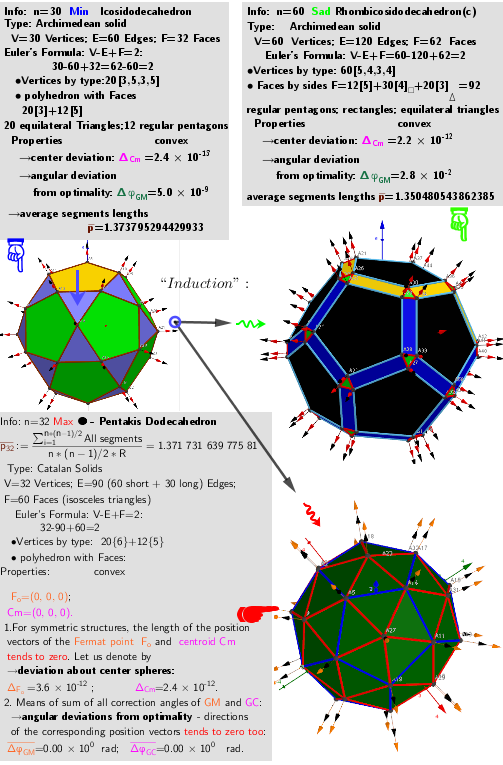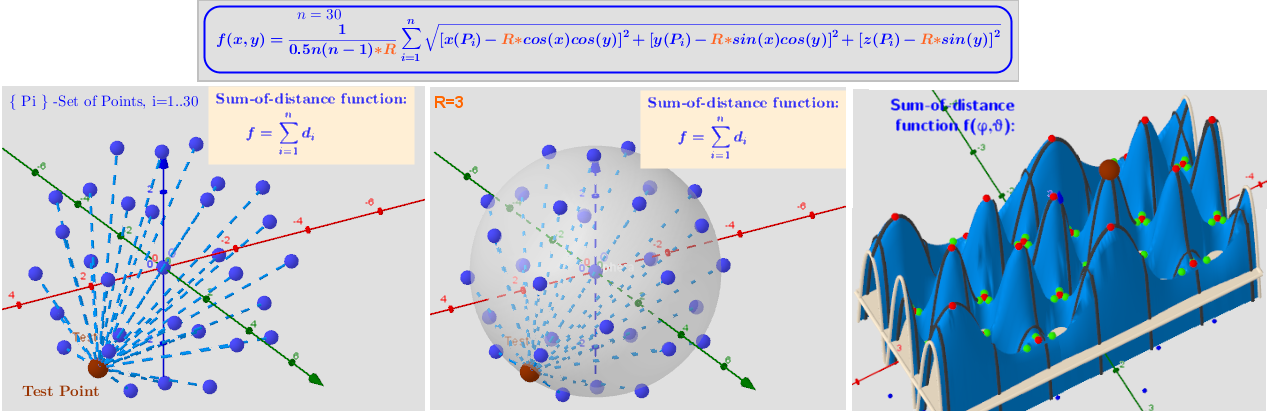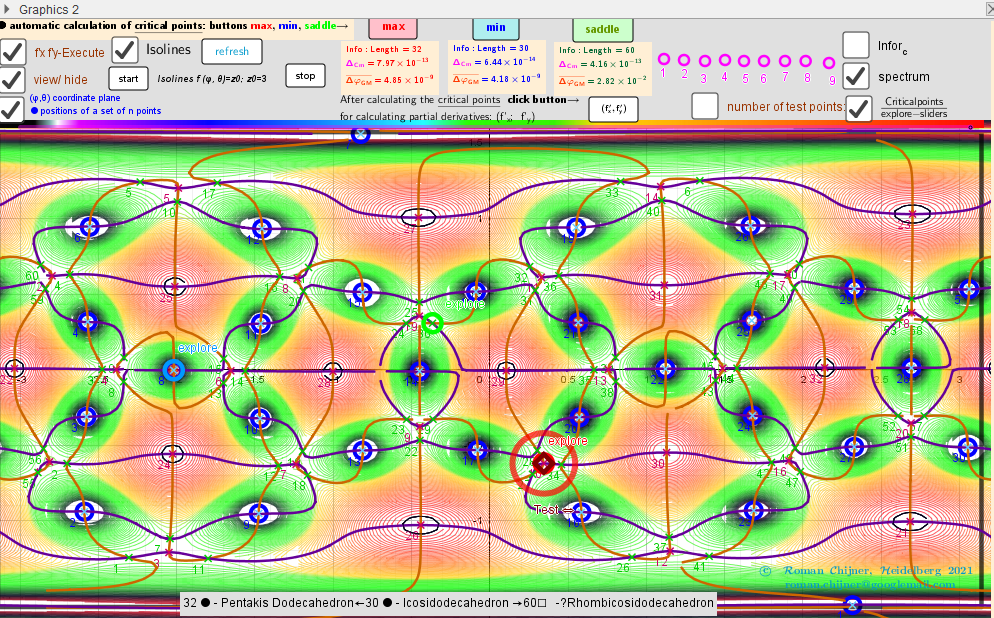# n=30 Icosidodecahedron. Images: A critical points scheme for Generating uniformly distributed points on a sphere.

The applet illustrates the case where 30 vertices of a Icosidodecahedron "induce" the vertices of two other polyhedra: V=32 ●Pentakis Dodecahedron← V=30 ●Icosidodecahedron →V=60 ☐Rhombicosidodecahedron. Generating polyhedra is in https://www.geogebra.org/m/hymcebuw. Description are in https://www.geogebra.org/m/y8dnkeuu and https://www.geogebra.org/m/rkpxwceh.A system of points on a sphere S of radius R “induces” on the sphere S0 of radius R0 three different sets of points, which are geometric medians (GM) -local maxima, minima and saddle points sum of distance function f(x). The angular coordinates of the spherical distribution of a system of points - local minima coincide with the original system of points.A uniform distribution of points on the surface of a sphere induces two other uniform distributions. Two-variable  function f(φ,θ) over a rectangular region: - π ≤φ ≤ π; -π/2≤θ≤π/2.Distribution of points Pi, test Point, Max/min/saddle -Critical points on a sphere. Vectors ∇f and ∇g at these points. max: Pentakis Dodecahedron min: Icosidodecahedron sad: Rhombicosidodecahedron(c)

## IsolinesIntersection points of implicit functions over a rectangular region: - π ≤φ ≤ π; -π/2≤θ≤π/2. A Test point -color indicator of the critical pointIsolines and Intersection points of implicit functions over a rectangular region: - π ≤φ ≤ π; -π/2≤θ≤π/2.﻿